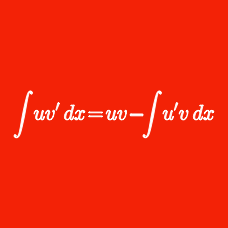Calculus

# Integration U-substitution - Problem Solving

When $a=2$ and $b=4,$ $\int_{a}^{b}{\sqrt{(x-a)(b-x)}}dx$ is equal to $\underline{\qquad}.$

For $n$ a positive integer, what is the indefinite integral $\int{ x{(1-x)}^{n+6} }dx?$

Details and assumptions

Use $C$ as the constant of integration.

Evaluate $\int_{0}^{\sqrt{3}} (x+4)^2 e^{x^2} dx+\int_{\sqrt{3}}^{0} (x-4)^2 e^{x^2} dx.$

Given that $\displaystyle \int_0^4 x^3\sqrt{9+x^2} dx = a$, what is the value of $\lfloor a \rfloor$?

Details and assumptions

Greatest Integer Function: $\lfloor x \rfloor: \mathbb{R} \rightarrow \mathbb{Z}$ refers to the greatest integer less than or equal to $x$. For example $\lfloor 2.3 \rfloor = 2$ and $\lfloor -3.4 \rfloor = -4$.

Determine the value of $100 \int_0^{\pi} \sqrt{ 1 + \sin x} \, dx$.

This problem is proposed by Cody.

×